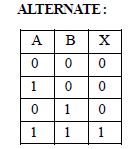Courses

# Test: Semiconductor Electronics 1 - From Past 28 Years Questions

## 25 Questions MCQ Test Physics Class 12 | Test: Semiconductor Electronics 1 - From Past 28 Years Questions

Description
This mock test of Test: Semiconductor Electronics 1 - From Past 28 Years Questions for Class 12 helps you for every Class 12 entrance exam. This contains 25 Multiple Choice Questions for Class 12 Test: Semiconductor Electronics 1 - From Past 28 Years Questions (mcq) to study with solutions a complete question bank. The solved questions answers in this Test: Semiconductor Electronics 1 - From Past 28 Years Questions quiz give you a good mix of easy questions and tough questions. Class 12 students definitely take this Test: Semiconductor Electronics 1 - From Past 28 Years Questions exercise for a better result in the exam. You can find other Test: Semiconductor Electronics 1 - From Past 28 Years Questions extra questions, long questions & short questions for Class 12 on EduRev as well by searching above.
QUESTION: 1

### Sodium has body centred packing. Distancebetween two nearest atoms is 3.7 Å. The latticeparameter is 

Solution: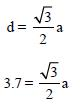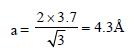QUESTION: 2

### Which one of the following statement isFALSE ?    

Solution:

Majority carriers in an n-type semiconductor
are electrons.

QUESTION: 3

### Which one of the following bonds produces asolid that reflects light in the visible region andwhose electrical conductivity decreases withtemperature and has high melting point?   

Solution:

For a metal, conductivity decreases with
increase in temperature.
Also, metal has high melting point.

QUESTION: 4

The device that can act as a complete electroniccircuit is 

Solution:

Integrated circuit can act as a complete
electronic circuit.

QUESTION: 5

A common emitter amplifier has a voltage gainof 50, an input impedance of 100Ω and anoutput impedance of 200Ω. The power gain ofthe amplifier is 

Solution:

Power gain = voltage gain × current gain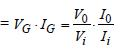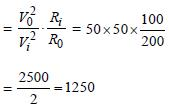QUESTION: 6

To get an output Y = 1 from the circuit shown below, the input must be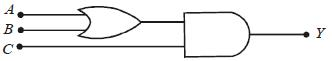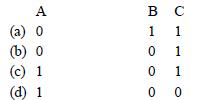Solution:

solution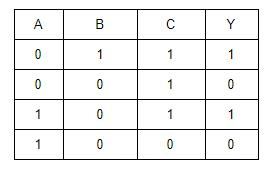answer is 'a'

QUESTION: 7

For transistor action :
(1) Base, emitter and collector regions should have similar size and doping concentrations.
(2) The base region must be very thin and lightly doped.
(3) The eimtter-base junction is forward biased and base-collector junction is reverse based.
(4) Both the emitter-base junction as well as the base-collector junction are forward biased.

Solution:

For transistor action, the base region must
be very thin and lightly doped. Also, the
emitter-base junction is forward biased and
base-collector junction is reverse biased

QUESTION: 8

The following Figure shows a logic gate circuit with two inputs A and B and the output Y. The voltage waveforms of A, B and Y are given :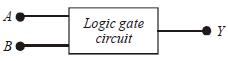The logic gate is :

Solution:

From the given waveforms, the truth table
is as follows.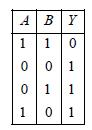The above truth table is for NAND gate.
Therefore, the logic gate is NAND gate.

QUESTION: 9

A transistor is operated in common emitter configuration at VC = 2V such that a change inthe base current from 100 μA to 300 μA produces a change in the collector current from 10mA to 20 mA. The current gain is 

Solution:

The current gain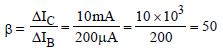QUESTION: 10

In forward biasing of the p–n junction 

Solution:

In forward biasing of the p-n junction, the
positive terminal of the battery is connected
to p-side and the negative terminal of the
battery is connected to n-side. The depletion
region becomes thin.

QUESTION: 11

Symbolic representation of four logic gate are shown as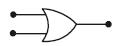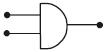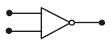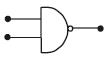Pick out which ones are for AND, NAND and NOT gates, respectively

Solution:
QUESTION: 12

If a small amount of antimony is added togermanium crystal 

Solution:

When small amount of antimony
(pentavalent) is added to germanium crystal then crystal becomes n-type semi conductor. Therefore, there will be more free electrons than holes in the semiconductor.

QUESTION: 13

In the following figure, the diodes which are forward biased, are [2011M]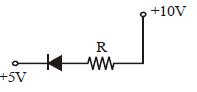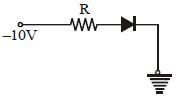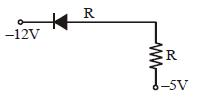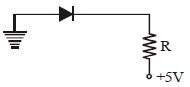Solution:

Only in (A) and (C) diodes are forward biased
as p-type should be at higher potential and
n-type at lower potential.

QUESTION: 14

Pure Si at 500K has equal number ofelectron (ne) and hole (nh) concentrations of1.5 × 1016 m–3. Doping by indium increases nhto 4.5 × 1022 m–3. The doped semiconductor isof [2011M]

Solution: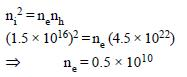or ne = 5 × 109
Given nh = 4.5 × 1022
⇒nh >> ne
∴ Semiconductor is p-type and
ne = 5 × 109 m–3.

QUESTION: 15

A zener diode, having breakdown voltage equal to 15V, is used in a voltage regulator circuit shown in figure. The current through the diode is [2011M]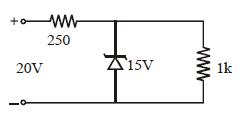Solution:

Voltage across zener diode is constant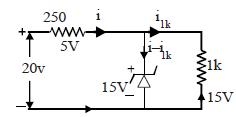Current in 1kΩ resistor,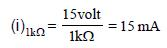Current in 250Ω resistor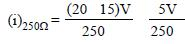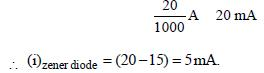QUESTION: 16

Two ideal diodes are connected to a battery as shown in the circuit. The current supplied by the battery is :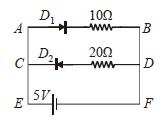Solution:

Here D1 is in forward bias and D2 is in
reverse bias so, D1 will conduct and D2 will
not conduct. Thus, no current will flow
through DC.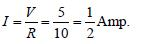QUESTION: 17

In a CE transistor amplifier, the audio signalvoltage across the collector resistance of 2kΩ is 2V. If the base resistance is 1kΩ and thecurrent amplification of the transistor is 100, theinput signal voltage is : 

Solution: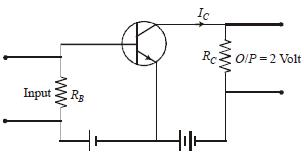The output voltage, across the load RC
V0 = IC RC = 2
The collector current (IC)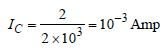Current gain (β)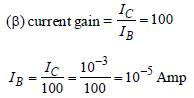Input voltage (Vi)
Vi = RB IB = 1 × 10, × 10–5 = 10–2 Volt
Vi = 10 mV

QUESTION: 18

C and Si both have same lattice structure, having 4 bonding electrons in each. However, C isinsulator whereas Si is intrinsic semiconductor.This is because : 

Solution: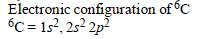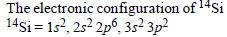As they are away from Nucleus, so effect
of nucleus is low for Si even for Sn and Pb
are almost mettalic.

QUESTION: 19

Transfer characteristics [output voltage (V0) vs input voltage (V1)] for a base biased transistor in CE configuration is as shown in the figure. For using transistor as a switch, it is used :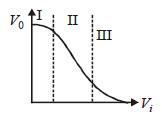Solution:

I → ON
II → OFF
In IInd state it is used as a amplifier it is
active region

QUESTION: 20

The figure shows a logic circuit with two inputs A and B and the output C. The voltage wave forms across A, B and C are as given. The logic circuit gate is :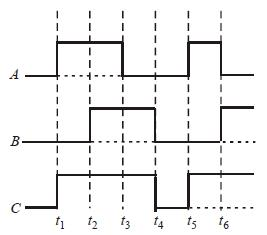Solution: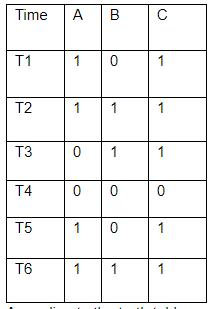According to the truth table we can clearly see that it is an OR Gate. Hence A is correct.

QUESTION: 21

The input resistance of a silicon transistor is100 Ω. Base current is changed by 40 μA which results in a change in collector current by 2 mA.This transistor is used as a common emitter amplifier with a load resistance of 4 KΩ. The voltage gain of the amplifier is : [2012M]

Solution: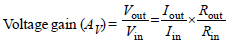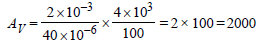QUESTION: 22

To get an output Y = 1 in given circuit which of the following input will be correct : [2012M]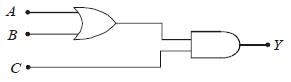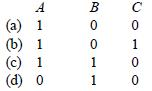Solution:

When A = 1, B = 0, C = 1 then Y = 1
y1 = 1 + 0 = 1
y = y1C = 1.1 = 1

QUESTION: 23

In a n-type semiconductor, which of the followingstatement is true? [NEET 2013]

Solution:

In a n-type semiconductor holes are
minority carriers and pentavalent atoms
are dopants.

QUESTION: 24

In a common emitter (CE) amplifier having a voltage gain G, the transistor used has transconductance 0.03 mho and current gain 25. If the above transistor is replaced with another one with transconductance 0.02 mho and current gain 20, the voltage gain will be   [NEET 2013]

Solution: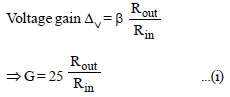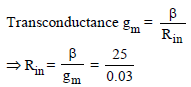Putting this value of Rin in eqn. (i)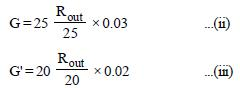From eqs. (ii) and (iii)
Voltage gain of new transistor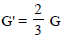QUESTION: 25

The output(X) of the logic circuit shown in figure will be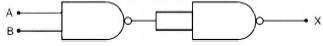Solution: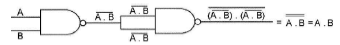i.e., output X = A.B